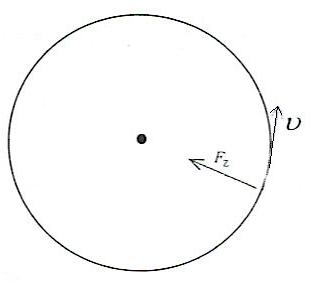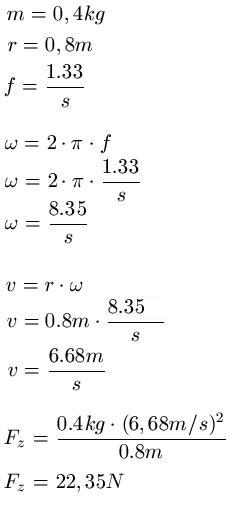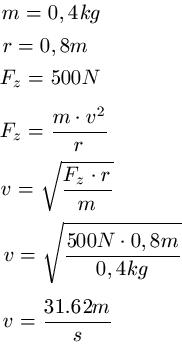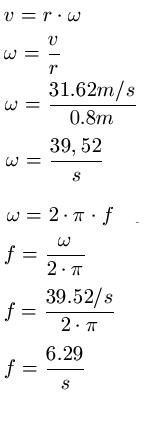# How do you find the centripetal acceleration

## Circular motion and centripetal force (physics)

The circular motion and the centripetal force are the subject of this article. We will explain important terms, what they are and how to do some calculations with the corresponding formulas.Everyone knows a circular movement, for example from a carousel. If you sit down, you will be driven in a circle at the same speed over a longer period of time. In physics, such a circular path is often represented as follows (we explain the terms below):Show:

### Circular motion definitions

Before I give you a few formulas, you should first know a few definitions of circular motion. There are:

• "v" is the path speed
• "T" is the period, i.e. the time for one revolution of the object
• "f" is the frequency, this is the number of revolutions per second of the object
• "ω" is the angular frequency, the circumferential angle per time in radians (formula follows)
Show:

### Formulas for circular motion

Calculate angular frequency:

• Formula: ω = 2 · π · f
• "ω" is the angular frequency per second [1 / s]
• "π" is the circle number, π = 3.14159
• "f" is the frequency per second [1 / s]

Calculate speed:

• Formula: v = r ω
• "v" is the speed in meters per second [m / s]
• "r" is the radius of the circle in meters [m]
• "ω" is the angular frequency per second [1 / s]

Calculate acceleration:

• Formula: a = v2 : r
• "a" is the acceleration in meters per second square [m / s2 ]
• "v" is the speed in meters per second [m / s]
• "r" is the radius of the circle in meters [m]

### centripetal force

In the case of a uniform circular movement, a force always acts on the body, which always points to the center of the circle. This force is called the centripetal force.

Calculate centripetal force:

• Formula: FZ = m · v2 : r
• "F.Z"is the centripetal force in Newtons [N]
• "m" is the mass of the object in kilograms [kg]
• "v" is the speed in meters per second [m / s]
• "r" is the radius of the circle in meters [m]

### Examples: centripetal force / circular motion

In the following we want to look at some examples that demonstrate how to use the formulas.

example 1: A 0.4kg body is thrown on a 0.8m long cord on a circular path. The frequency is f = 1.33 / s. What is the force?Example 2: A 0.4kg body is thrown on a 0.8m long cord on a circular path. The cord can withstand 500N load. At what number of revolutions does the cord break?Left:

### Who's Online

We have 1342 guests online